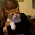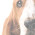## Wednesday, September 26, 2007

### NCLB Assessment Quality

The following problem is a released test item for fifth grade students, assessing their fourth grade achievement. It is from the New England Common Assessment Program (NECAP) Student Practice Test Booklet for Grade 5 Mathematics. Can you find the problem that I have with it? I used this problem with my fifth grade class today for their review. Testing begins next week. What are the standards for testing validity for high stakes tests?Technorati tags:
_/\_/\_

1.Could it be that when triangle equals zero, the statement is false?

2.Hi, pann. I had not even considered that possibility. I had several students give D as the answer because, they reasoned, 8÷1=8. That would make the triangle equal 1 and the square equal to 8. However, the defining equation would then be 8*8=1.

This contradiction makes D the wrong answer. We spent a lot of time discussing the importance of the first equation and how they have to read carefully, using the given information. So my objection to the question is its readability.

But you have raised another problem with the question that interests me. Zero was not excluded from consideration. Does this invalidate the question?

3.